# fvtool

Visualize frequency response of DSP filters

## Syntax

``fvtool(sysobj)``
``fvtool(sysobj,options)``
``fvtool(____,Name,Value)``

## Description

````fvtool(sysobj)` displays the magnitude response of the filter System object™.```

example

````fvtool(sysobj,options)` displays the response that is specified by the options.For example, to visualize the impulse response of an FIR filter System object, set `options` to `'impulse'`.Fs = 96e3; filtSpecs = fdesign.lowpass(20e3,22.05e3,1,80,Fs); firlp2 = design(filtSpecs,'equiripple','SystemObject',true); fvtool(firlp2,'impulse');```
````fvtool(____,Name,Value)` visualizes the response of the filter with each specified property set to the specified value.For more input options, see FVTool in Signal Processing Toolbox™.```

## Examples

collapse all

Create two lowpass halfband decimation filters for data sampled at 44.1 kHz. The design method in the first filter is set to `"Equiripple" `and in the second filter is set to `"Kaiser"`.

The output data rate is 1/2 the input sample rate, or 22.05 kHz. Specify the filter order to be 52 with a transition width of 4.1 kHz.

```Fs = 44.1e3; filterspec = "Filter order and transition width"; Order = 52; TW = 4.1e3; firhalfbanddecimEqui = dsp.FIRHalfbandDecimator(... Specification=filterspec,... FilterOrder=Order,... TransitionWidth=TW,... DesignMethod="Equiripple",... SampleRate=Fs); firhalfbanddecimKaiser = dsp.FIRHalfbandDecimator(... Specification=filterspec,... FilterOrder=Order,... TransitionWidth=TW,... DesignMethod="Kaiser",... SampleRate=Fs);```

Plot the impulse response of both the filters. The zeroth-order coefficient is delayed 26 samples, which is equal to the group delay of the filter. This yields a causal halfband filter.

```hfvt = fvtool(firhalfbanddecimEqui,firhalfbanddecimKaiser,... Analysis="impulse"); legend(hfvt,{'Equiripple','Kaiser'})```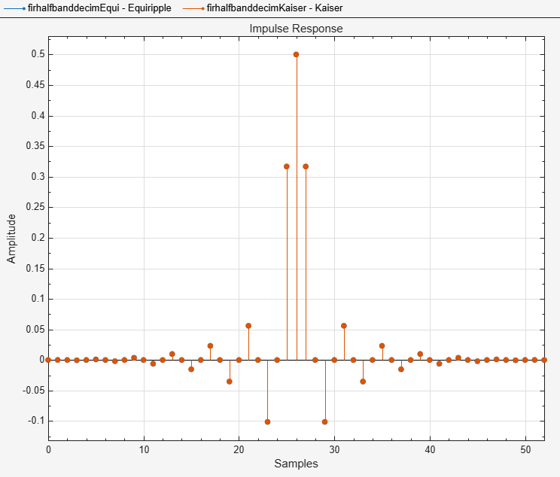Plot the magnitude and phase response.

If the filter specifications are tight, say a very high filter order with a very narrow transition width, the filter designed using the `"Kaiser"` method converges more effectively.

```hvftMag = fvtool(firhalfbanddecimEqui,firhalfbanddecimKaiser,... Analysis="Magnitude"); legend(hvftMag,{'Equiripple','Kaiser'})```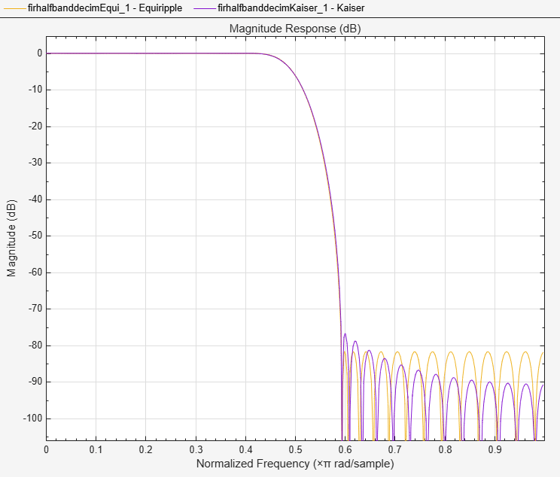```hvftPhase = fvtool(firhalfbanddecimEqui,firhalfbanddecimKaiser,... Analysis="Phase"); legend(hvftPhase,{'Equiripple','Kaiser'})```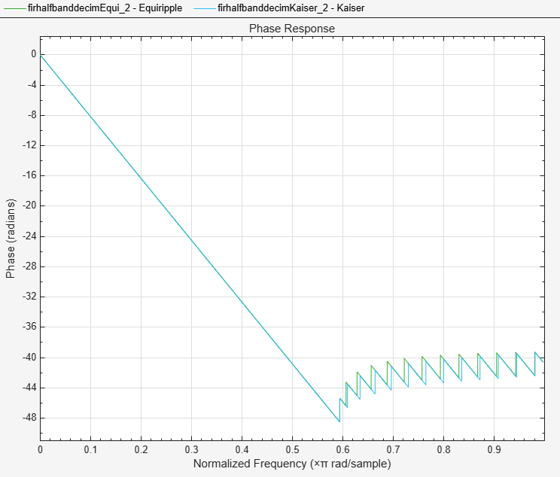Create a minimum-order FIR lowpass filter for data sampled at 44.1 kHz. Specify a passband frequency of 8 kHz, a stopband frequency of 12 kHz, a passband ripple of 0.1 dB, and a stopband attenuation of 80 dB.

```Fs = 44.1e3; filtertype = 'FIR'; Fpass = 8e3; Fstop = 12e3; Rp = 0.1; Astop = 80; FIRLPF = dsp.LowpassFilter(SampleRate=Fs,... FilterType=filtertype,... PassbandFrequency=Fpass,... StopbandFrequency=Fstop,... PassbandRipple=Rp,... StopbandAttenuation=Astop);```

Design a minimum-order IIR lowpass filter with the same properties as the FIR lowpass filter. Change the `FilterType` property of the cloned filter to `IIR`.

```IIRLPF = clone(FIRLPF); IIRLPF.FilterType = 'IIR';```

Plot the impulse response of the FIR lowpass filter. The zeroth-order coefficient is delayed by 19 samples, which is equal to the group delay of the filter. The FIR lowpass filter is a causal FIR filter.

`fvtool(FIRLPF,Analysis='impulse')`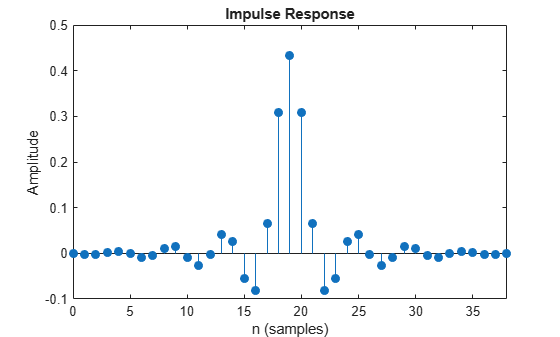Plot the impulse response of the IIR lowpass filter.

`fvtool(IIRLPF,Analysis='impulse')`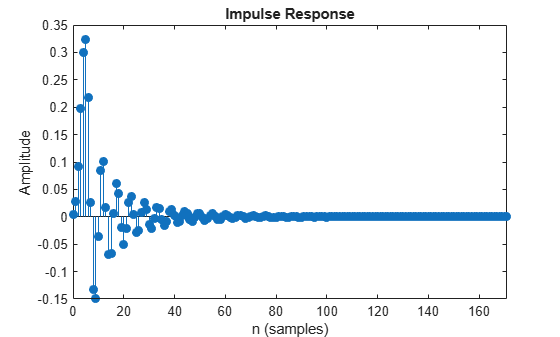Plot the magnitude and phase response of the FIR lowpass filter.

`fvtool(FIRLPF,Analysis='freq')`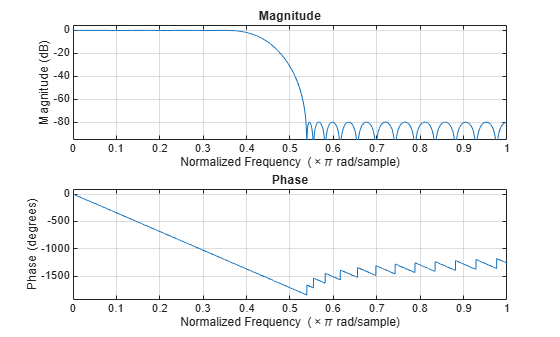Plot the magnitude and phase response of the IIR lowpass filter.

`fvtool(IIRLPF,Analysis='freq')`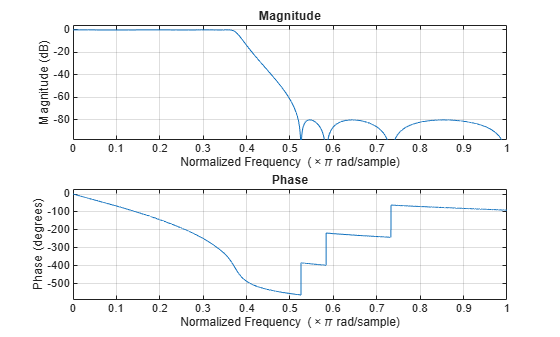Calculate the cost of implementing the FIR lowpass filter.

`cost(FIRLPF)`
```ans = struct with fields: NumCoefficients: 39 NumStates: 38 MultiplicationsPerInputSample: 39 AdditionsPerInputSample: 38 ```

Calculate the cost of implementing the IIR lowpass filter. The IIR filter is more efficient to implement than the FIR filter.

`cost(IIRLPF)`
```ans = struct with fields: NumCoefficients: 18 NumStates: 14 MultiplicationsPerInputSample: 18 AdditionsPerInputSample: 14 ```

Calculate the group delay of the FIR lowpass filter.

`grpdelay(FIRLPF)`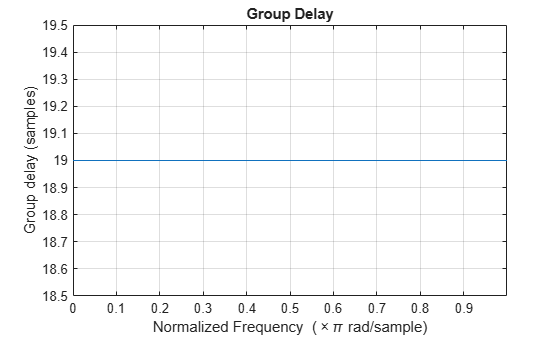Calculate the group delay of the IIR lowpass filter. The FIR filter has a constant group delay (linear phase), while its IIR counterpart does not.

`grpdelay(IIRLPF)`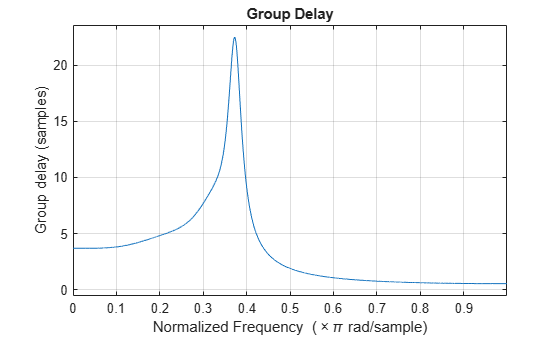## Input Arguments

collapse all

Input filter, specified as one of the following filter System objects:

Example: ```firFilt = dsp.FIRFilter('Numerator', fir1(130, 2000/(8000/2))); fvtool(firFilt)```

Filter analysis options, specified as one of the following:

• `'magnitude'` –– Magnitude response

• `'phase'` –– Phase response

• `'freq'` –– Frequency response

• `'grpdelay'` –– Group delay

• `'phasedelay'` –– Phase delay

• `'impulse'` –– Impulse response

• `'step'` –– Step response

• `'polezero'` –– Pole zero plot

• `'coefficients'` –– Coefficients vector

• `'info'` –– Filter information

• `'magestimate'` –– Magnitude response estimate

• `'noisepower'` –– Round-off noise power spectrum

Example: `fvtool(firFilt,'freq')`

### Name-Value Arguments

Specify optional pairs of arguments as `Name1=Value1,...,NameN=ValueN`, where `Name` is the argument name and `Value` is the corresponding value. Name-value arguments must appear after other arguments, but the order of the pairs does not matter.

Before R2021a, use commas to separate each name and value, and enclose `Name` in quotes.

Example: ```firFilt = dsp.FIRFilter('Numerator', fir1(130, 2000/(8000/2))); fvtool(firFilt,'Arithmetic','single')```

Sampling rate, specified as a scalar. This value determines the Nyquist interval [-Fs/2 Fs/2] in which the fvtool shows the frequency response of the filters in the channelizer.

Data Types: `single` | `double`

Specify the arithmetic used during analysis. The analysis tool assumes a double-precision filter when the arithmetic input is not specified and the filter System object is unlocked. The `'Arithmetic'` property set to `'Fixed'` applies only to filter System objects with fixed-point properties.

When the `'Arithmetic'` property is set to `'Fixed'`, the tool shows both the double-precision reference filter and the quantized version of the filter. The `CoefficientsDataType` property in the respective filter System object is used in creating the quantized version of the filter for all the analyses options except for the two below:

• `'magestimate'` –– Magnitude response estimate.

• `'noisepower'` –– Round-off noise power spectrum

For these two analyses options, all the fixed-point settings are used in analyzing the quantized version of the filter.

## Version History

Introduced before R2006a